# Electric Circuit

An electric circuit is a representation of a real circuit that is used to connect different devices. Electric circuits have various components. We need to learn about all these components to understand the electrical circuit and the flow of current in it.

Alessandro Volta was the first to come up with the idea of an electric circuit in 1800. Volta discovered that by connecting bowls of salt solution with metal strips, he could create a continuous flow of electricity. Later, he made his voltaic pile out of alternating discs of copper, zinc, and cardboard that had been soaked in a salt solution (an early battery). By connecting a wire from top to bottom, he was able to make the electric current flow across the circuit.

## Electric Circuit

Closed loops or paths, in which the current flows is called electric circuit. In electric circuits, various components are added that performs various task. In other words, we can say that an electric circuit is a collection of elements designed in such a way that they carry electric current in a specific direction. The source is the starting point from which the current starts and the return are the points where it ends.

The diagram of the electric circuit is given below, let’s learn about the electric circuit through the diagram.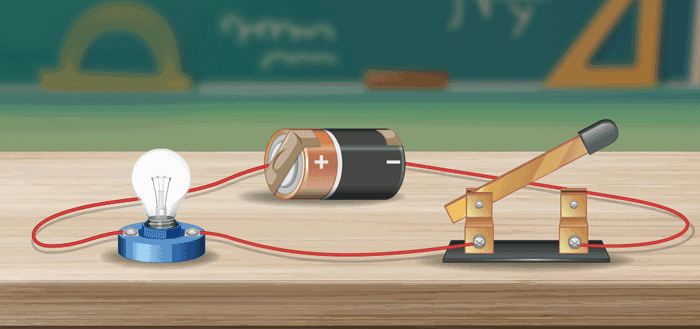The above electric circuit has,

• Electric bulb
• Wire(Conductor)
• A battery

If we connect one end of the battery (say positive end) to the positive terminal of the bulb we observe that nothing happens that the bulb does not list up but as soon as we connect the other end of the battery with the bulb and the circuit is completed the bulb list up. Thus, it is clear that current only flows in an electric circuit if it is closed and such circuits are called complete circuits.

If all the connections are not secured in a circuit it may cause a fire in the circuit which can not be extinguished by normal methods. Water to extinguish the electric fire is strictly prohibited as it can cause electric shock.

## Types of Electric Circuits

Many types of electrical circuits are used in our daily life some of the most common electric circuits are,

• Open Circuit
• Closed Circuit
• Short Circuit
• Series Circuit
• Parallel Circuit

### Open Circuit

A circuit in which the switch is open and current does not flow through the circuit is called an open circuit.

The image given below shows an open circuit.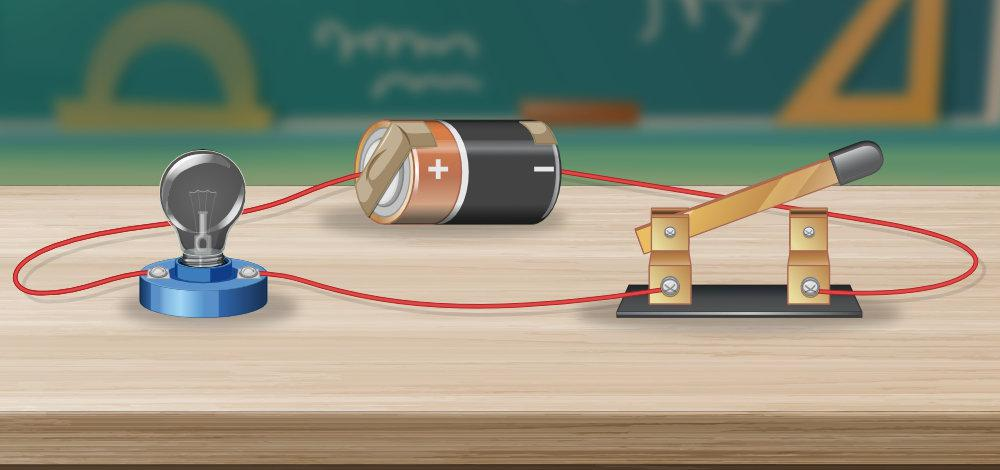### Closed Circuit

A circuit in which the switch is closed and current flows through the circuit is called an open circuit.

The image given below shows a closed circuit.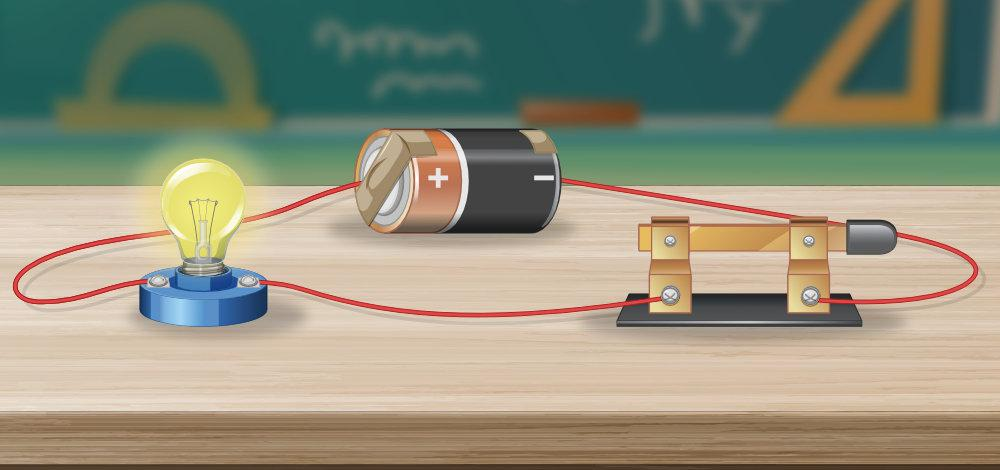### Short Circuit

Whenever the current in the circuit follows a shorter path rather than following its usual path short circuit happens.

The image given below shows a short circuit.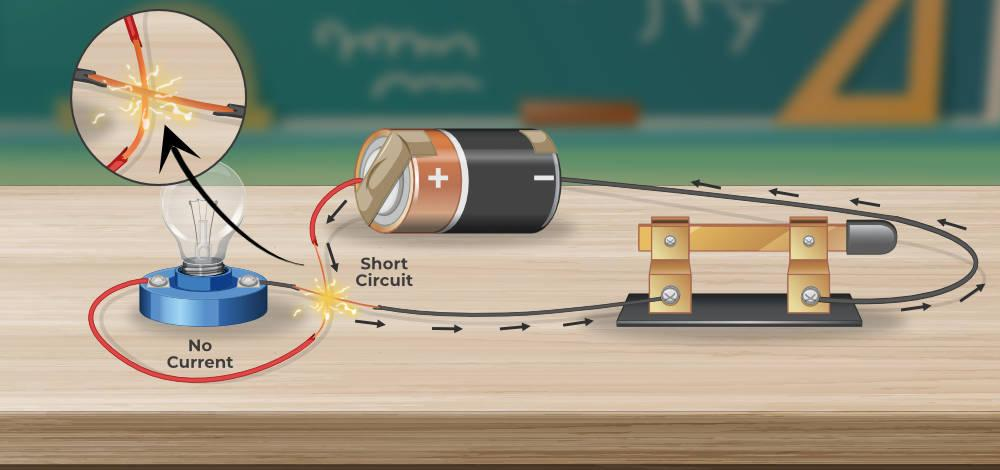The consequence of the short circuit is that the electric board or the electric system catches the fire. The major cause of the electric fire is the short circuit. The image below shows the fire caused by the short circuit.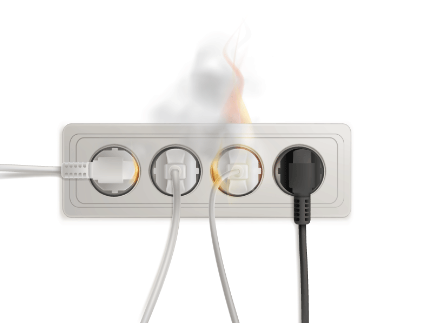### Series Circuit

If all the resistance are connected in the series combination we say that it is a series circuit.

The image given below shows a series circuit.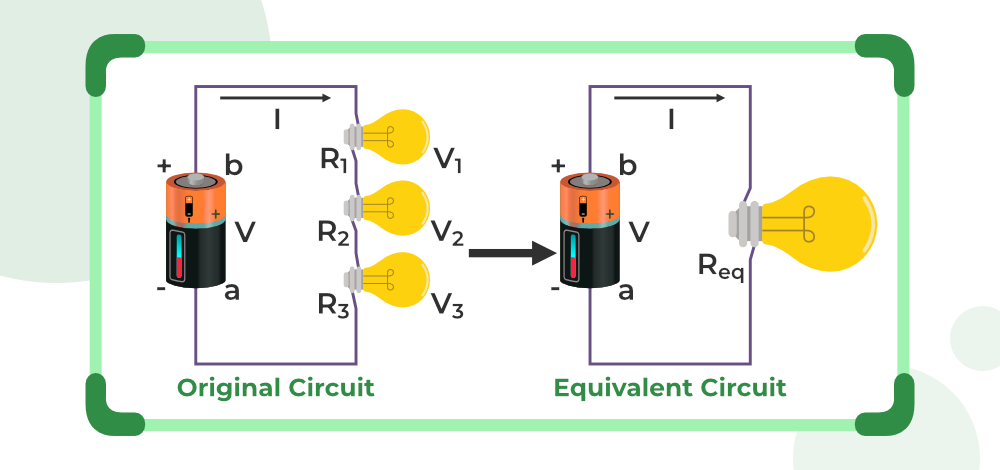### Parallel Circuit

If all the resistors are connected in a parallel combination we say that it is a parallel circuit.

The image given below shows a parallel circuit.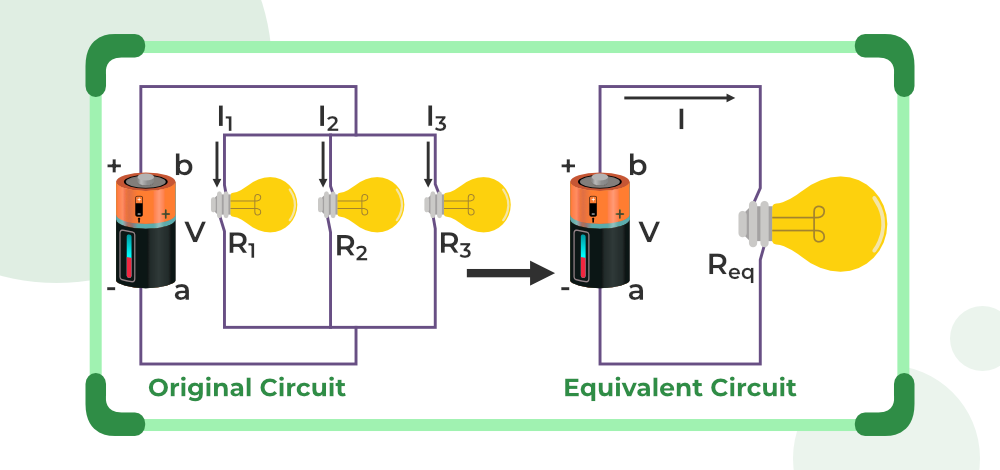For more, Series and Parallel Resistance

## Domestic Electric Circuit

The circuit used in our home is called a Domestic circuit and is specifically made for domestic purposes. Various components of the domestic circuit are,

Mains Supply: Electric power we receive in our houses is called the main supply.

Cables: Electric power is supplied by cables which are either open or underground.

There are three types of wires used in domestic circuits which are

• Earth Wire
• Live Wire
• Neutral Wire

Potential Difference (or Voltage): In our country, the current is supplied at 220V in domestic circuits.

Electric current is passed to our house at 220 volts through a circuit called a Fuse. If the supplied voltage varies a lot as compared to the allowed voltage the fuse wire gets to break and stopped the flow of current in the circuit. The current then passes through the meter board.

From the meter board generally, two types of current are supplied to the circuit.

• 15 Amp current: This current is used by appliances with higher power ratings, like geysers, ACs, refrigerators, electric motors, and others.
• 5 Amp current: This current is used by appliances with lower power ratings, like TV, fans, bulbs, computers, smartphones, and others.

## Electric Circuit Symbols

To draw electric circuits various symbols are used some of the important symbols that are used in physics for drawing electric  symbols are,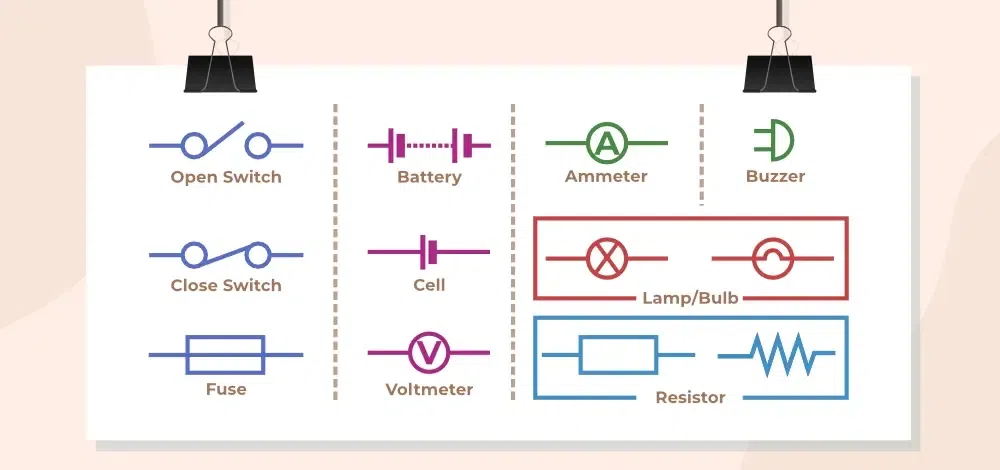## Simple Circuit

A circuit that consists of a power source, conductors, switch, and load is a simple circuit. A simple circuit does not have various components and some of the basic components are,

The image given below shows a simple circuit.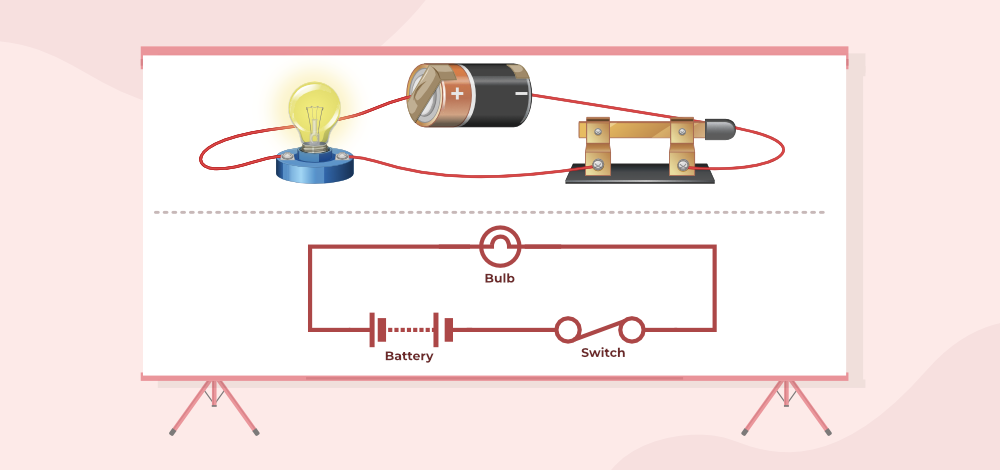• Cell: It is the power source that provides the potential difference in the electric circuit.
• Load: A resistor that consumes current in a circuit is called the load. Generally, a load is a bulb.
• Conductors: Copper conductors that conduct electricity in a circuit are called conductors.
• Switch: A device that controls the incoming flow of current in an electric circuit is called a switch.

## Electric Circuit Formula

Various formulas that are used in solving electric circuits are,

## FAQs on Electric Circuits

### Q1: What is electric current?

The rate of flow of electric charges is used to express electric current. The quantity of charge moving across a specific area in a given amount of time is referred to as the rate of flow.

I = Q/t

where
I represents electric current,
Q represents net charge,
t represents time in seconds.

### Q2: What is Electric Potential?

Electric potential is the amount of effort required to move a positive charge from one location to another.

### Q3: Who invented the first electric circuit?

Alessandro Volta invented the first electric circuit in 1800.

### Q4: What can cause the current to cease flowing in an Electric Circuit?

Electric current flows only when there is a continuous connection from a battery to various components and back to the battery, any disruption will cause the current to cease flowing.

### Q5: What is an Electric Circuit?

A closed loop or path designed to flow the current is called an Electric Circuit. It is generally made to give electrical energy.

### Q6: What is a Switch?

A device that controls the flow of the current in an electric circuit is called a switch.

### Q7: What is the formula to calculate Electric Current?

The current in the electric circuit is calculated by the formula,

I = Q/t

where,
I is the current
Q is the charge flowing
t is the time period

Whether you're preparing for your first job interview or aiming to upskill in this ever-evolving tech landscape, GeeksforGeeks Courses are your key to success. We provide top-quality content at affordable prices, all geared towards accelerating your growth in a time-bound manner. Join the millions we've already empowered, and we're here to do the same for you. Don't miss out - check it out now!

Previous
Next